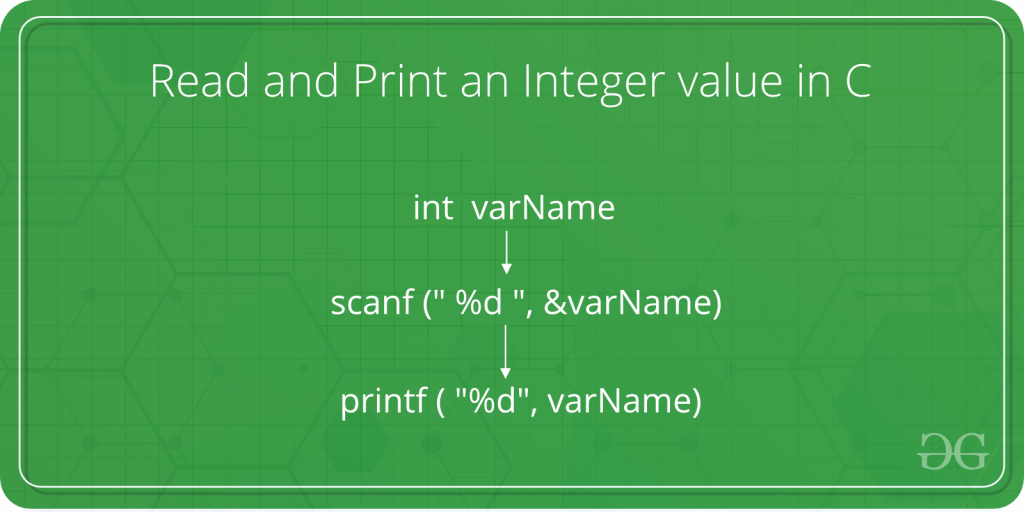# How to Read and Print an Integer value in C

• Difficulty Level : Medium
• Last Updated : 05 Dec, 2018

The given task is to take an integer as input from the user and print that integer in C language.In below program, the syntax and procedures to take the integer as input from the user is shown in C language.

Steps:

1. The user enters an integer value when asked.
2. This value is taken from the user with the help of scanf() method. The scanf() method, in C, reads the value from the console as per the type specified.

Syntax:

```scanf("%X", &variableOfXType);

where %X is the format specifier in C
It is a way to tell the compiler
what type of data is in a variable

and

& is the address operator in C,
which tells the compiler to change the
real value of this variable, stored at this
address in the memory.
```
3. For an integer value, the X is replaced with type int. The syntax of scanf() method becomes as follows then:

Syntax:

```scanf("%d", &variableOfIntType);
```
4. This entered value is now stored in the variableOfIntType.
5. Now to print this value, printf() method is used. The printf() method, in C, prints the value passed as the parameter to it, on the console screen.

Syntax:

```printf("%X", variableOfXType);
```
6. For an integer value, the X is replaced with type int. The syntax of printf() method becomes as follows then:

Syntax:

```printf("%d", variableOfIntType);
```
7. Hence, the integer value is successfully read and printed.

Program:

## C

 `// C program to take an integer``// as input and print it`` ` `#include `` ` `int` `main()``{`` ` `    ``// Declare the variables``    ``int` `num;`` ` `    ``// Input the integer``    ``printf``(``"Enter the integer: "``);``    ``scanf``(``"%d"``, &num);`` ` `    ``// Display the integer``    ``printf``(``"Entered integer is: %d"``, num);`` ` `    ``return` `0;``}`

Output:

```Enter the integer: 10
Entered integer is: 10
```
My Personal Notes arrow_drop_up